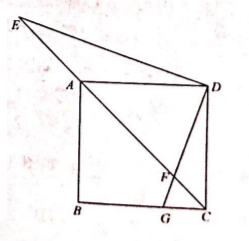$\text{A.}$ $2 \sqrt{2}$ $\text{B.}$ $\frac{4 \sqrt{5}}{3}$ $\text{C.}$ $\frac{9}{2}$ $\text{D.}$ $\frac{3 \sqrt{5}}{2}$
【答案】 D